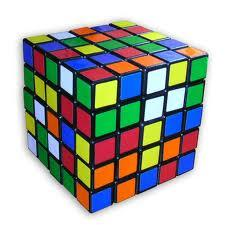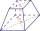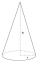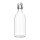# Transforming cuboid

Cuboid with dimensions 6 cm, 10, and 11 cm is converted into a cube with the same volume. What is its edge length?

Correct result:

x =  8.7 cm

#### Solution:We would be very happy if you find an error in the example, spelling mistakes, or inaccuracies, and please send it to us. We thank you!Tips to related online calculators
Looking for a statistical calculator?
Do you want to convert length units?
Tip: Our volume units converter will help you with the conversion of volume units.

## Next similar math problems:

• Concrete columnThe concrete column of the highway bridge has the shape of a block with dimensions of 1m x 0.8m x 25m. It should be lifted by crane to a height of 20m. What is the power of his engine if the lifting takes 2 minutes?
• Into boxHow many cubes with an edge of 2.5 cm fit into a box measuring 11.6 cm; 8.9 cm and 13.75 cm?
• FlowerpotThe block-shaped flowerpot has external dimensions: length 1.25 m, width 10 cm and height 11 cm. The thickness of the boards from which it is made is 0.8 cm. How many liters of soil is needed to fill it 1 cm below the top edge? What surface do we have to
• Cargo spaceThe cargo space of the truck has the dimensions a = 4.2 m, b = 1.9 m, c = 8.1 dm. Calculate its volume.
• Cuboid diagonalsThe cuboid has dimensions of 15, 20 and 40 cm. Calculate its volume and surface, the length of the body diagonal and the lengths of all three wall diagonals.
• The woodenThe wooden block measures 12 cm, 24 cm, and 30 cm. Peter wants to cut it into several identical cubes. At least how many cubes can he get?
• AquariumHow many liters of water can fit in an aquarium measuring 30, 15 and 20 cm?
• AngieAngie poured 4 cartons of milk, each with 2.4 litres, into a mixing bowl. How many litres of milk were there altogether?
• Two vasesMichaela has two vases in her collection. The first vase has the shape of a cone with a base diameter d = 20 cm; the second vase has the shape of a truncated cone with the diameter of the lower base d1 = 25 cm and with the diameter of the upper base d2 =
• Sphere cutA sphere segment is cut off from a sphere k with radius r = 1. The volume of the sphere inscribed in this segment is equal to 1/6 of the volume of the segment. What is the distance of the cutting plane from the center of the sphere?
• Triangular pyramidCalculate the volume of a regular triangular pyramid with edge length a = 12cm and pyramid height v = 20cm.
• Pentagonal pyramidFind the volume and surface of a regular pentagonal pyramid with a base edge a = 12.8 cm and a height v = 32.1 cm.
• Wooden bowls20 wooden bowls in the shape of a truncated cone should be painted on the outside and inside with wood varnish. We need 0.1 l of paint to paint 200 cm2. How many liters of paint do we have to buy if the bowls are 25 cm high, the bottom of the bowl has a d
• From plasticineMichael modeled from plasticine a 15 cm high pyramid with a rectangular base with the sides of the base a = 12 cm and b = 8 cm. From this pyramid, Janka modeled a rotating cone with a base diameter d = 10 cm. How tall was Janka's cone?
• ZhiweiZhiwei wants to divide 19 litres of water equally into 6 pots. Find the volume of water in each pot?
• Fresh juiceThe seller offers fresh-squeezed juice, which the customer either pours into his own containers or sells it in liter plastic bottles, which the customer buys from him. A liter of juice costs 40 CZK more than a bottle. There is no charge for turning the ju
• Find theFind the surface area of a regular quadrilateral pyramid which has a volume of 24 dm3 and a height of 45 cm.